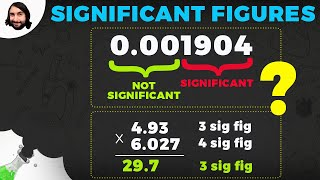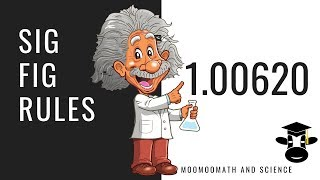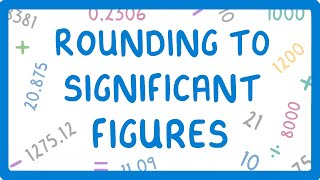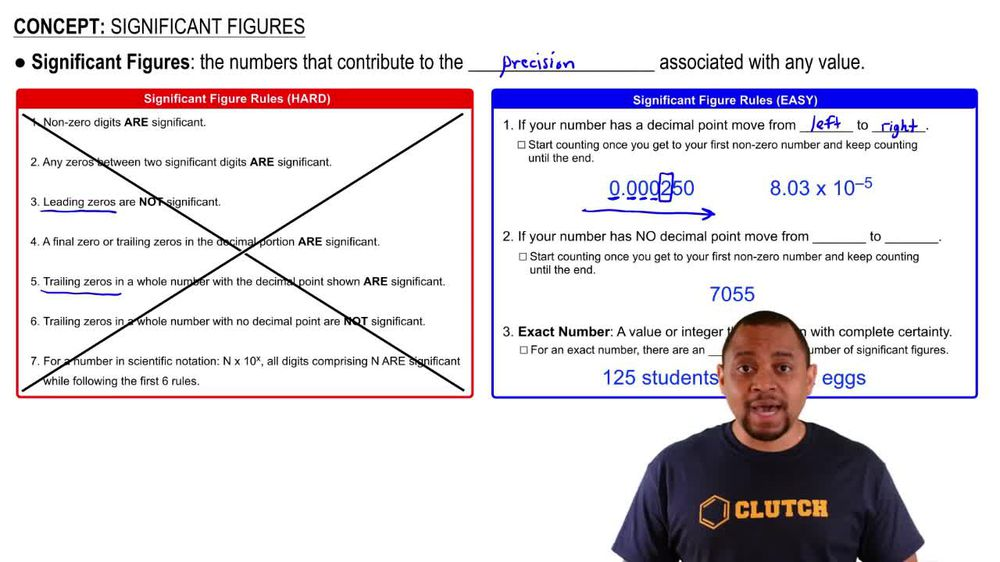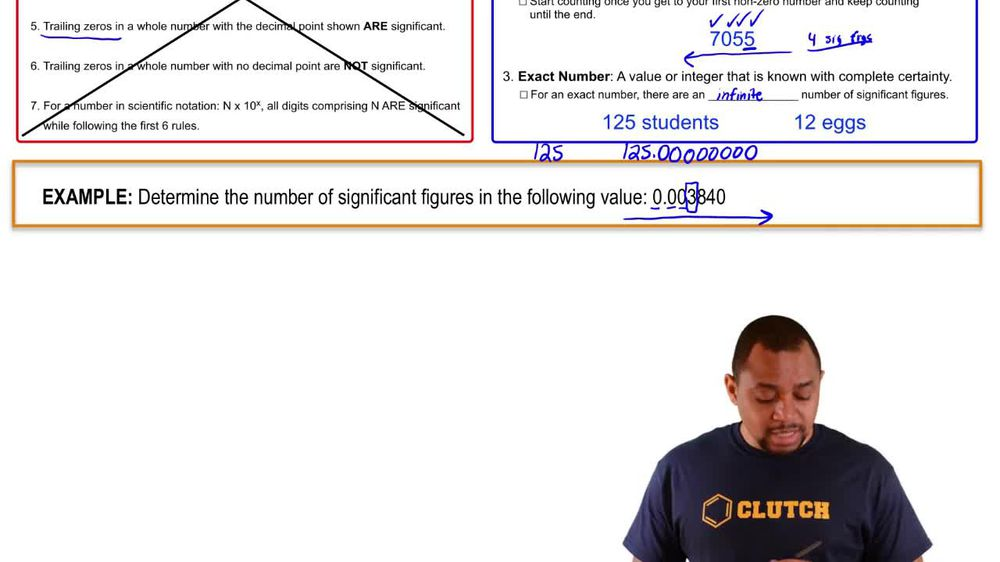Start typing, then use the up and down arrows to select an option from the list.
1. 1. Intro to General Chemistry2. Significant Figures
Problem

# How many significant figures are in each of the following measurements? (a) 35.0445 g

Relevant Solution1m
Play a video:
Hello everyone. Today. We are being asked to determine if the following statement is true or false. There are more significant figures in 0. than and 0.00088. So The first thing you wanna do is you want to analyze each number. So first we'll do 0.088 when it comes to significant figures. Any whole number greater than zero can be accounted for as a stick fig. So therefore this first number has to significant figures. As for the 2nd 1, 0.00088, we once again have two non zero integers. Therefore we have two significant figures as well. Therefore the answer is false as each of these numbers have the same number of significant figures, which is to I hope this helped and until next time.